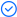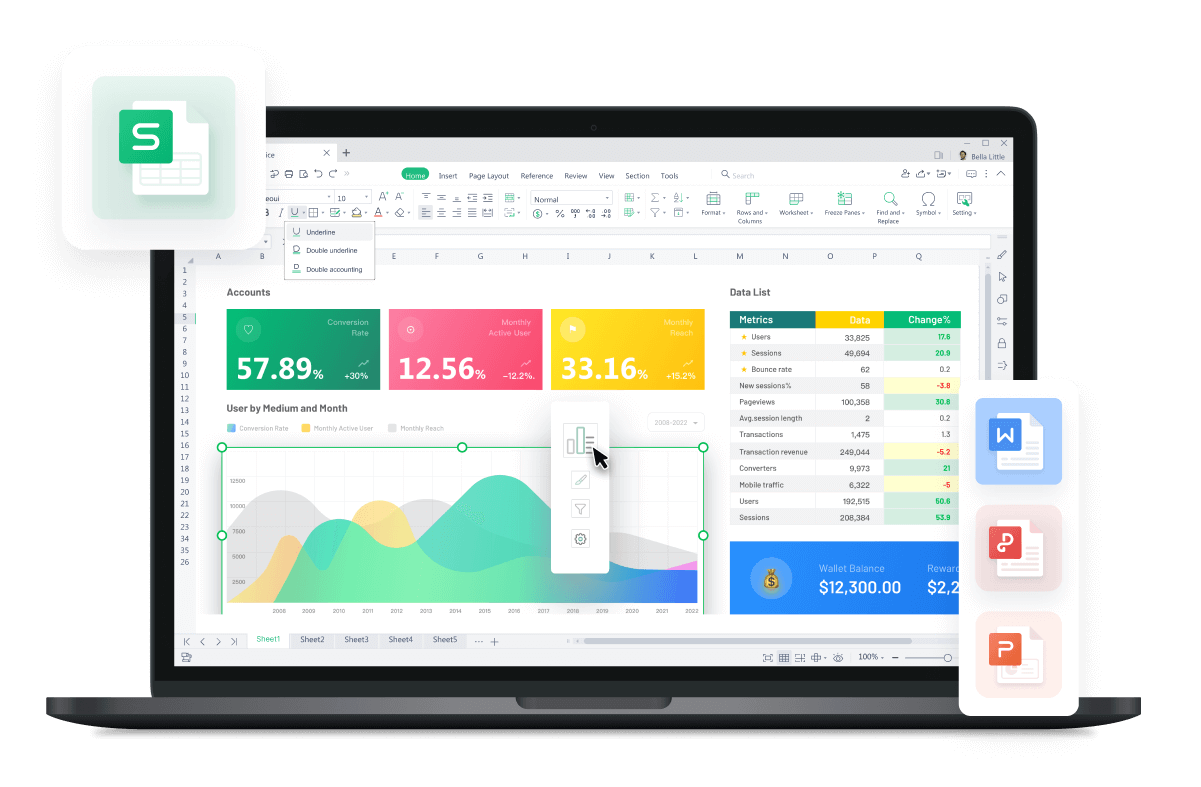WPS Office

Free All-in-One Office Suite with PDF EditorRead, edit, and convert PDFs with the powerful PDF toolkit.Microsoft-like interface, easy to use.

Windows • MacOS • Linux • iOS • Android# INDEX MATCH formulasINDEX MATCH formulas

In the previous videos, we've learned about the usages of INDEX and MATCH formulas, respectively.

The INDEX function can return a value in a cell regarding its column and row number in an array. Combining the INDEX MATCH formulas makes it flexible in value lookup. We can break the limitations of VLOOKUPand realize the reverse lookup.

Here is a form recording the personal information of staff.How can we look up the 'Number'of a corresponding staff?

We can run the INDEX MATCH formulas.

1. First, we need to locate the row of 'Name'and the column of 'Number'.

2. Enter the formula =MATCH(H11,F2:F39,0).The result will be '2',which means Robert's 'Number' is located in the second row of the selected array.

3. Enter =MATCH(H12,A1:F1,0) in Cell I12.The result will be'1',which means the value'Robert'is located in the first columnof cell range A1:F1.

In the previous steps, the two MATCH functions indicated the column and row of the lookup values regarding their column or row number within the array. In the latter part of this video, we'll combine these two functions with INDEX for further value lookup.

1. Select cell J3 and enter the combined formulas, which is shown below. The core of the formula is INDEX, with two MATCH functions respectively in the second and third parameters.

* Note: When combining the two functions, we should use an absolute cell referenceto avoid mistakes by the referred cell.

2. Press Enter and drag to use the AutoFill function. In this way, we can get the expected result.

For the tutorials of the MATCH formula and the INDEX formula, please visit WPS Academy.